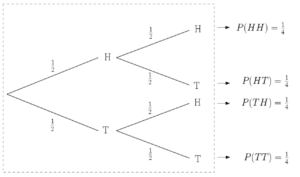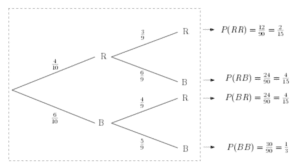# Tree Diagrams

Tree Diagrams, like Venn Diagrams,  provide a simple visual way of representing events and their associated probabilities. They can be used to calculate more complicated probabilities. Tree Diagrams display the probabilities different event outcomes on the ‘branches’ of the diagram. Typically, the events are considered sequentially (one after the other) moving along the branches from left to right.

## Independent Events in Tree Diagrams

Consider the very simple case of flipping a coin twice – each toss is a separate event. If the coin is fair, the probability of tossing heads and tails is clearly 0.5. In the second toss, these probabilities still hold.In this example, all sets of branches have identical probabilities. This is typical of a tree diagram where the events are independent (learn about Independent Events).

We can see from the tree diagram that the probability of tossing two tails in a row, i.e. P(TT), is 1/4. Note that to specify that two event outcomes occur, we move along the branches and so we must multiply the probabilities on the branches together (one outcome AND another outcome implies MULTIPLY). One outcome OR another implies addition. For example, suppose we want to know the probability that at least one head was thrown in two coin tosses. In this case, we can have HH, HT or TH and so we add their associated probabilities together. It follows that the probability of tossing at least one head is 3/4. This makes sense since the only way in which we don’t toss at least one head is if we toss no heads, i.e. two tails.

## Conditional Probability on Tree Diagrams

If the probabilities on the second set of branches were different, there is dependence on the outcome of the first event. This is known as conditional probability.Consider the slightly more complicated example of drawing counters from a bag without replacement. The bag starts off with 4 red counters and 6 blue counters. Two counters are drawn from the bag one after the other.
We can see from this diagram, for example, that the probability of drawing one of each is:

$P(RB)+P(BR)=\frac{4}{15}+\frac{4}{15}=\frac{8}{15}.$

## Tree Diagram Examples

Three fair dice are rolled simultaneously. Find the probability that at least one dice has a number less than or equal to 2.

A false positive diagnosis in medical testing is a positive test result when the disease IS NOT present. Similarly, a false negative is one in which a medical test gives a negative result when the disease IS present. Click here for more details.

2% of people are living with a given condition. The chances of a screening procedure for the condition giving a positive result when the condition is present is 95%. There is a 0.5% chance that the screen diagnoses the condition when it is NOT present. Calculate the probability that the screening procedure gives a correct diagnosis.

…coming soon.